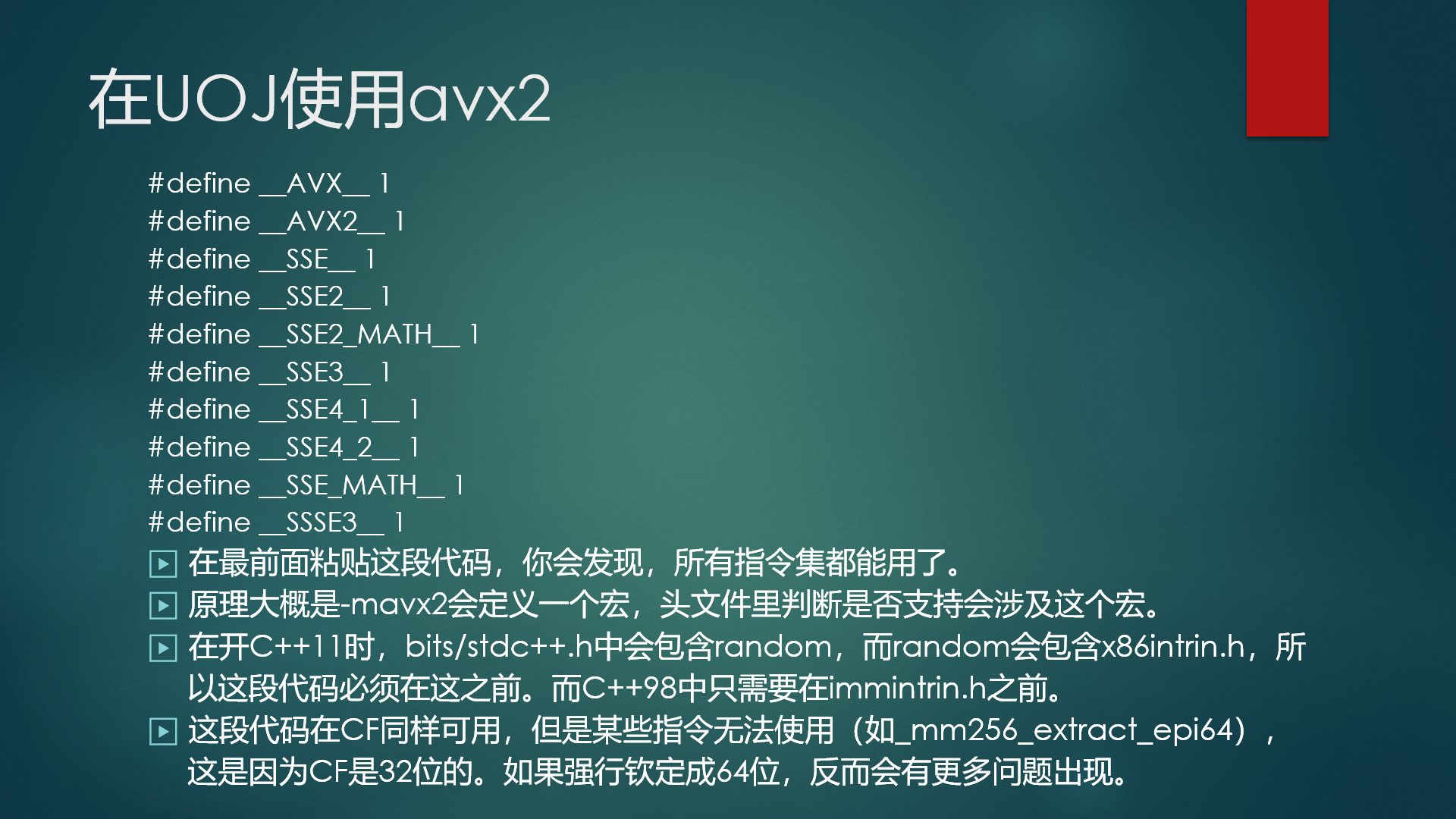# ouuan 的博客# ouuan 的博客

### n方过百万 暴力碾标算——指令集优化的基础使用

posted on 2019-02-01 16:53:36 | under 洛谷日报 |

ok不扯了，进入正题...

# 适用范围

## 环境sse2，avx 什么的都是指令集的名字。

# 具体使用

## pragma&include

#pragma GCC optimize("Ofast,no-stack-protector,unroll-loops,fast-math")
#pragma GCC target("sse,sse2,sse3,ssse3,sse4.1,sse4.2,avx,avx2,popcnt,tune=native")

#include <immintrin.h>
#include <emmintrin.h>

## 指令使用

__m256i _mm256_set_epi32 (int e7, int e6, int e5, int e4, int e3, int e2, int e1, int e0)：参数是八个数，也就是一个“分块”里的数，注意是逆序的。返回值是一个含这八个数的“分块”。

__m256i _mm256_set_epi64x (__int64 e3, __int64 e2, __int64 e1, __int64 e0)：和上面一样，只不过是 $64$ 位整数，也就是 long long。

__m256i _mm256_set1_epi32 (int a)：相当于 _mm256_set_epi32(a,a,a,a,a,a,a,a)

__m256i _mm256_add_epi32 (__m256i a, __m256i b)：把两个“分块”的对应位置分别相加，返回结果。

__m256i _mm256_cmpeq_epi32 (__m256i a, __m256i b)：判断两个“分块”的对应位置是否相等，若相等则返回的“分块”对应位置是 0xffffffff，否则是 0

__m256i _mm256_cmpgt_epi32 (__m256i a, __m256i b)：和上面一样，只不过比较符是大于而不是相等。

__m256i _mm256_and_si256 (__m256i a, __m256i b)：返回两个“分块”的按位与，可以配合上面两条比较指令来使用。

## 访问数据

#include <cstdio>

#pragma GCC optimize("Ofast,no-stack-protector,unroll-loops,fast-math")
#pragma GCC target("sse,sse2,sse3,ssse3,sse4.1,sse4.2,avx,avx2,popcnt,tune=native")

#include <immintrin.h>
#include <emmintrin.h>

typedef __m256i oak;

oak a;

int main()
{
a=_mm256_set_epi32(1,2,3,4,5,6,7,8);
printf("%d",a);
return 0;
}

a=_mm256_set_epi32(1,2,3,4,5,6,7,8);
int *b=(int *)&a;
printf("%d",b);

#include <cstdio>

#pragma GCC optimize("Ofast,no-stack-protector,unroll-loops,fast-math")
#pragma GCC target("sse,sse2,sse3,ssse3,sse4.1,sse4.2,avx,avx2,popcnt,tune=native")

#include <immintrin.h>
#include <emmintrin.h>

typedef __m256i oak;

int A,*b;
oak a,x;

int main()
{
int i;

for (i=0;i<80;++i) scanf("%d",A+i);

for (i=0;i<10;++i) a[i]=_mm256_set_epi32(A[i*8+7],A[i*8+6],A[i*8+5],A[i*8+4],A[i*8+3],A[i*8+2],A[i*8+1],A[i*8]);

b=(int *)&a;

x=_mm256_set1_epi32(233);

for (i=0;i<80;++i) printf("%d ",b[i]);

return 0;
}

# 例题

【模板】线段树1，这题其实是最简单的，然而由于 dl 出题人把值域搞到了 long long，常数只能除以四，需要卡卡常，多提交几次才能过。

[Ynoi2018]五彩斑斓的世界，神司怒艹lxl标算的课件例题。

Simple Tree，这个还要树剖，只不过也还好，神司是直接内嵌汇编写的，没有测过用函数能不能过..

#include <cstdio>

#pragma GCC optimize("Ofast,no-stack-protector,unroll-loops,fast-math")
#pragma GCC target("sse,sse2,sse3,ssse3,sse4.1,sse4.2,avx,avx2,popcnt,tune=native")

#include <immintrin.h>
#include <emmintrin.h>

const int N=1000010;

typedef __m256i oak;

int n,m,tot,*a;
oak A[N>>3];
char op;

void modify(int l,int r,int x)
{
while ((l&7)&&l<r) a[l++]+=x; //处理左边不是整块的部分，和分块的处理方法是一样的
if (l==r) return;
while (r&7) a[--r]+=x; //处理右边不是整块的部分
if (l==r) return;
oak t=_mm256_set1_epi32(x); //剩下的部分整块加上x
}

int query(int l,int r,int x)
{
int out=0;
while ((l&7)&&l<r) out+=int(a[l++]>=x); //处理左边不是整块的部分
if (l==r) return out;
while (r&7) out+=int(a[--r]>=x); //处理右边不是整块的部分
if (l==r) return out;
oak t=_mm256_set1_epi32(1); //这个1是每个大于等于x的数的贡献
oak ans=_mm256_set1_epi32(0); //这个ans是用来存答案的
oak cp=_mm256_set1_epi32(x-1); //这个是用来比较的，题目中是大于等于，所以和x-1比较
for (l>>=3,r>>=3;l<r;++l) ans=_mm256_add_epi32(ans,_mm256_and_si256(t,_mm256_cmpgt_epi32(A[l],cp))); //这个意会一下，作用是数当前块有几个大于x-1的数
for (int i=0;i<4;++i) out+=(ans[i]&0xffffffff)+(ans[i]>>32); //最后统计答案，因为ans[i]是一个long long，所以要前32位和后32位分别统计
return out;
}

int main()
{
int i,l,r,x,aa;

scanf("%d%d",&n,&m);

while (n) //读入比较鬼畜，需要每次读8个数
{
if (n<8)
{
for (i=0;i<n;++i) scanf("%d",aa+i);
n=0;
}
else
{
for (i=0;i<8;++i) scanf("%d",aa+i);
n-=8;
}
A[tot++]=_mm256_set_epi32(aa,aa,aa,aa,aa,aa,aa,aa);
}

a=(int*)&A;

while (m--)
{
scanf("%s%d%d%d",op,&l,&r,&x);
if (op=='M') modify(l-1,r,x);
else printf("%d\n",query(l-1,r,x));
}

return 0;
}